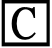### Home > AC > Chapter 16 > Lesson 16.9.1.2 > Problem9-30

9-30.Challenge: Your bank offers an interest rate of $4.88%$ on savings and says that the APR (annual percentage rate) is $5.00%$; that is, if I invest $100$, after one year, my bank account will have $105$ in it. Assume the bank rounds correctly to two decimal places.

1. What is the minimum whole number of times the bank compounds my interest?

$20$ times

2. What is the maximum number of times the bank compounds my interest?

There is no max, continuing compounding will still round to $5.00\%$.

3. Assuming the bank is giving exactly $5\%$ APR (no rounding), how many times per year is the account compounded.

Use Guess and Check.